# 1,90 % p.a. is the maximal interest rate under the term deposits for 1 year10.02.2020

## Bankchart.com.au has surveyed the terms of term deposits for 1 year in the national currency. The survey covered all the deposits with any form of interests repayment, regardless of the replenishment option or the deposit partial withdrawal option. Our website's analysts have studied the loan offers from all Australian banks. Review date - 10.02.2020

As of 25 January 2020 the deposits under review have been offered by 33 banks.  The average interest rate under such deposits equals 1,28 % p.a. The total number of the deposits programs available for the specified terms is 129.

The maximal return among the deposits under review is offered by ING Direct. The interest rate under "Personal Term Deposit" deposit program constitutes 1,90 % p.a. The table below shows the best offers by each bank selected by Bankchart.com.au (in terms of profitability) for the aforementioned terms.

### The TOP-33 term deposits for 1 year, as of 25 January 2020

#

Name

Interest Rate, %

1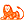ING Direct

«Personal Term Deposit»

1,90

interest rate, %

rate type

fixed

interest periodicity

at maturity

minimum sum

\$ 10 000,00

2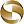Mega International Commercial Bank

«Term Deposits»

1,70

interest rate, %

rate type

fixed

interest periodicity

at maturity

minimum sum

\$ 1 000,00

3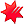National Australia Bank

«Farm Management Deposits - special rate»

1,70

interest rate, %

rate type

fixed

interest periodicity

at maturity, annually

minimum sum

\$ 10 000,00

4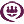RACQ

«Term Deposit»

1,70

interest rate, %

rate type

fixed

interest periodicity

quarterly

minimum sum

\$ 500,00

5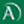Arab Bank

«Fixed Term Deposit»

1,65

interest rate, %

rate type

fixed

interest periodicity

at maturity

minimum sum

\$ 50 000,00

6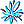AMP Bank

«Term Deposits»

1,65

interest rate, %

rate type

fixed

interest periodicity

at maturity

minimum sum

\$ 25 000,00

7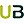Ubank

«Term deposit»

1,60

interest rate, %

rate type

variable

interest periodicity

at maturity

minimum sum

\$ 0,00

8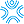Bank First

«Term Deposits»

1,60

interest rate, %

rate type

fixed

interest periodicity

monthly

minimum sum

\$ 50 000,00

9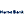Hume Bank

«Term Deposits»

1,60

interest rate, %

rate type

fixed

interest periodicity

at maturity, annually

minimum sum

\$ 5 000,00

10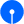State Bank of India

«Term Deposits»

1,60

interest rate, %

rate type

fixed

interest periodicity

at maturity

minimum sum

\$ 100,00

11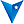BankVic

«Term deposit»

1,60

interest rate, %

rate type

fixed

interest periodicity

at maturity

minimum sum

\$ 50 000,00

12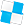Police Bank

«Term Deposits»

1,60

interest rate, %

rate type

fixed

interest periodicity

at maturity

minimum sum

\$ 10 000,00

13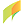G&C Mutual Bank

«Term Deposits»

1,55

interest rate, %

rate type

fixed

interest periodicity

at maturity, annually

minimum sum

\$ 1 000,00

14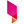Bank Australia

«Term Deposits»

1,55

interest rate, %

rate type

fixed

interest periodicity

at maturity

minimum sum

\$ 500,00

15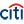Citibank

«Term deposit»

1,55

interest rate, %

rate type

fixed

interest periodicity

monthly

minimum sum

\$ 250 000,00

16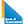Bank of Queensland

«Term deposit»

1,55

interest rate, %

rate type

fixed

interest periodicity

at maturity

minimum sum

\$ 5 000,00

17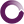Beyond Bank Australia

«Farm Management Deposit»

1,50

interest rate, %

rate type

fixed

interest periodicity

at maturity

minimum sum

\$ 200 000,00

18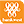Bank of Western Australia

«Online Term Deposit»

1,50

interest rate, %

rate type

fixed

interest periodicity

at maturity, annually

minimum sum

\$ 5 000,00

19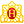Heritage Bank

«Term Deposits»

1,50

interest rate, %

rate type

fixed

interest periodicity

at maturity

minimum sum

\$ 1 000,00

20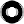Macquarie Bank

«Term Deposit»

1,45

interest rate, %

rate type

fixed

interest periodicity

monthly

minimum sum

\$ 0,00

21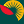Suncorp Bank

«Term Deposit»

1,45

interest rate, %

rate type

fixed

interest periodicity

at maturity

minimum sum

\$ 1 000 000,00

22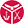Defence Bank

«RSA Term Deposit»

1,45

interest rate, %

rate type

fixed

interest periodicity

at maturity

minimum sum

\$ 10 000,00

23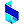Bank of Sydney

«Online Term Deposit»

1,35

interest rate, %

rate type

fixed

interest periodicity

at maturity

minimum sum

\$ 250 000,00

24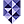Bank of Melbourne

«Term Deposits»

1,30

interest rate, %

rate type

fixed

interest periodicity

at maturity

minimum sum

\$ 1 000,00

25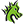St. George Bank

«Term Deposits»

1,30

interest rate, %

rate type

fixed

interest periodicity

at maturity

minimum sum

\$ 1 000,00

26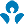Australia and New Zealand (ANZ)

1,30

interest rate, %

rate type

fixed

interest periodicity

every 6 months

minimum sum

\$ 5 000,00

27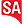Bank SA

«Term Deposits»

1,30

interest rate, %

rate type

fixed

interest periodicity

at maturity

minimum sum

\$ 1 000,00

28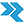Rural Bank

«Long Term Deposit»

1,25

interest rate, %

rate type

fixed

interest periodicity

annually

minimum sum

\$ 150 000,00

29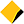Commonwealth Bank of Australia

«Term Deposits»

1,25

interest rate, %

rate type

fixed

interest periodicity

annually

minimum sum

\$ 50 000,00

30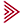Bendigo and Adelaide Bank

«Term deposit»

1,25

interest rate, %

rate type

fixed

interest periodicity

at maturity

minimum sum

\$ 5 000,00

31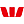Westpac Bank

«Term Deposit»

1,20

interest rate, %

rate type

fixed

interest periodicity

monthly

minimum sum

\$ 5 000,00

32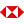HSBC Bank

«Term Deposits»

1,10

interest rate, %

rate type

fixed

interest periodicity

at maturity

minimum sum

\$ 5 000,00

33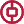Bank of China

«TERM DEPOSIT»

0,90

interest rate, %

rate type

fixed

interest periodicity

at maturity

minimum sum

\$ 20 000,00

To view the up-to-date rating of the deposits at our website here.